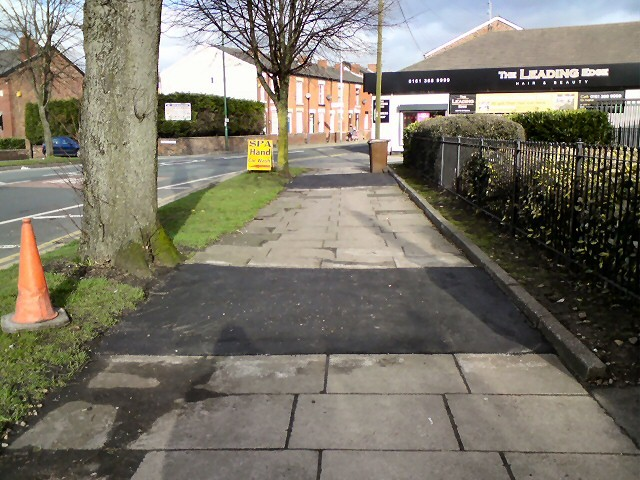# Beginners Guide To Concrete Maturity

Posted on August 27, 2019

## What Is Concrete Maturity?Pavement patches are just one of the many concrete applications where maturity testing is done.

Simply put, maturity is a real-time approach to estimating the compressive strength of in-place concrete, and relating it to the effects of temperature and time.

This process is used to measure the progression of the curing process; it is an accurate indexing method of figuring out the strength of the concrete, while it cures.

The maturity method, often just called maturity, carries out strength testing in a non-destructive manner, making it ideal for use by builders, suppliers, and subcontractors. It is the perfect way of conducting quick evaluations to determine the exact instant at which the concrete reaches its required strength.

Since maturity is directly related to the strength and durability of the concrete, this method is the best way to measure it without depending on standard laboratory testing, or using test specimens. It can virtually eliminate the use of concrete cylinder break testing, with the exception of breaking cylinders as a means of verification.

## How Is Concrete Maturity Measured?

The standard practice for measuring maturity is designated ASTMC1074. The method is defined as “a technique for estimating concrete strength that is based on the assumption that samples of a given concrete mixture attain equal strengths if they attain equal values of the maturity index.”

When concrete cures, it gives off heat energy that is directly proportional to the rate of curing. Through the maturity method, the subsequent rise in temperature can be recorded, and included in the report.

Since concrete mixes have differing strength-maturity relationships, the maturity method can be used to determine the strength of a particular kind of mix.

The maturity index value requires mix calibration to be implemented. It is the role of this calibration to define the exact relationship between the concrete’s strength, and maturity.

As a part of this process, data collection is carried out by wireless concrete maturity sensors. These sensors measure the temperature of the curing concrete, and compare them to the previously acquired calibrations to measure strength.

As an additional advantage, these sensors can be connected to any smart device, transmitting the data instantly to your medium of choice without the need for an expensive data logger.

### The Calibration Process

The ASTM C1074 standard recommends these steps be followed to achieve the calibrate mix:

1. The number of cylinders you use should at least be 17; 2 cylinders to monitor the temperature, while the others are to be utilized for breaks in the compression strength. Curing of all of these cylinders should be done at the same time, preferably in a temperature controlled area to ensure proper humidity (ASTM C511).
2. Decide on at least 5 different times to break, for example; 1, 4, 7, 15 and 28 days, to determine the compression strength of the two cylinders for each day. The third cylinder should only be broken if the final result deviation is greater than 10% from the mean value. Be sure to pay careful attention to the break times.
3. While breaking, calculate the maturity values of the two initial cylinders used to monitor the temperature — average out this value.
4. By now, you should have a minimum of 5 data values with specific strengths linked to particular maturity levels. This data can then be plotted in a graph to get a log equation curve.
5. The calibration curve obtained can be further validated by adding on a few cylinders to the subsequent pouring. When comparing the strength that is calculated from the data received from the lab, and the strength obtained from maturity, a difference of up to 10% is allowed.

## Key Benefits Of Concrete Maturity

The maturity method allows evaluation of concrete strength at a specific time – it is a precise and time sensitive way of measuring the strength of concrete.

Concrete Maturity leads to better quality control and assurance since there is minimal reliance on the preparation and testing of concrete cylinders. The proper care and handling of concrete cylinders is critical to the accuracy of strength testing results. Unfortunately, that aspect of concrete testing is sometimes overlooked. To learn more about concrete cylinder curing please click here.

## Applications Of Concrete Maturity Testing

Listed below are just a few of the various areas of application that benefit from the maturity method.

• High-rise buildings
• Parking garages
• Early formwork removal and fast cycle times
• Cast-in-place concrete construction
• Bridges
• Pavement patches
• Mass concrete
• Cold-weather and hot-weather concreting
• Chimneys and cooling towers
• Value engineering projects